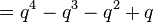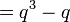# Endomorphism structure of projective general linear group of degree two over a finite field

## Contents

This article gives specific information, namely, endomorphism structure, about a family of groups, namely: projective general linear group of degree two. This article restricts attention to the case where the underlying ring is a finite field.
View endomorphism structure of group families | View other specific information about projective general linear group of degree two | View other specific information about group families for rings of the type finite field

This page describes the endomorphism structure of$PGL(2,q)$, the projective general linear group of degree two over a finite field of size a prime power$q$. We denote by$p$ the underlying prime of$q$, so$q = p^r$ where$r = \log_pq$.

## Particular cases

Group$p$$q$$r = \log_pq$ Order of the group$= q^4 - q^3 - q^2 + q$ Automorphism group Order of the automorphism group$= r(q^3 - q)$
Order of inner automorphism group ($= q^3 - q$) Order of outer automorphism group, same as the Galois group ($= r$) Endomorphism structure page
symmetric group:S3 2 2 1 6 symmetric group:S3 6 6 1 endomorphism structure of symmetric group:S3
symmetric group:S4 3 3 1 48 symmetric group:S4 24 24 1 endomorphism structure of symmetric group:S4
alternating group:A5 2 4 2 60 symmetric group:S5 120 60 2 endomorphism structure of alternating group:A5
symmetric group:S5 5 5 1 120 symmetric group:S5 120 120 1 endomorphism structure of symmetric group:S5
projective general linear group:PGL(2,7) 7 7 1 336 projective general linear group:PGL(2,7) 336 336 1 endomorphism structure of projective general linear group:PGL(2,7)
projective special linear group:PSL(2,8) 2 8 3 504 Ree group:Ree(3) 1512 504 3 endomorphism structure of projective special linear group:PSL(2,8)
projective general linear group:PGL(2,9) 3 9 2 720 automorphism group of alternating group:A6 1440 720 2 endomorphism structure of projective general linear group:PGL(2,9)

## Endomorphism structure

### Automorphism structure

Construct Value Order Comment
automorphism group projective semilinear group of degree two$P\Gamma L(2,q)$$r(q^3 - q)$ Semidirect product$PGL(2,q) \rtimes \operatorname{Aut}(\mathbb{F}_q)$ where$\operatorname{Aut}(\mathbb{F}_q) \cong \mathbb{Z}/r\mathbb{Z}$ is cyclic and generated by the Frobenius$x \mapsto x^p$.
inner automorphism group$PGL(2,q)$ itself$q^3 - q$ The group is a centerless group hence equals its inner automorphism group.
outer automorphism group$\operatorname{Aut}(\mathbb{F}_q) \cong \mathbb{Z}/r\mathbb{Z}$$r$ The outer automorphism group is trivial, and hence, the group is a complete group (note that it's already centerless) in the case$r = 1$, i.e., in the case that$q = p$. In other words, a projective general linear group of degree two over a finite field is complete if and only if that field is a prime field.